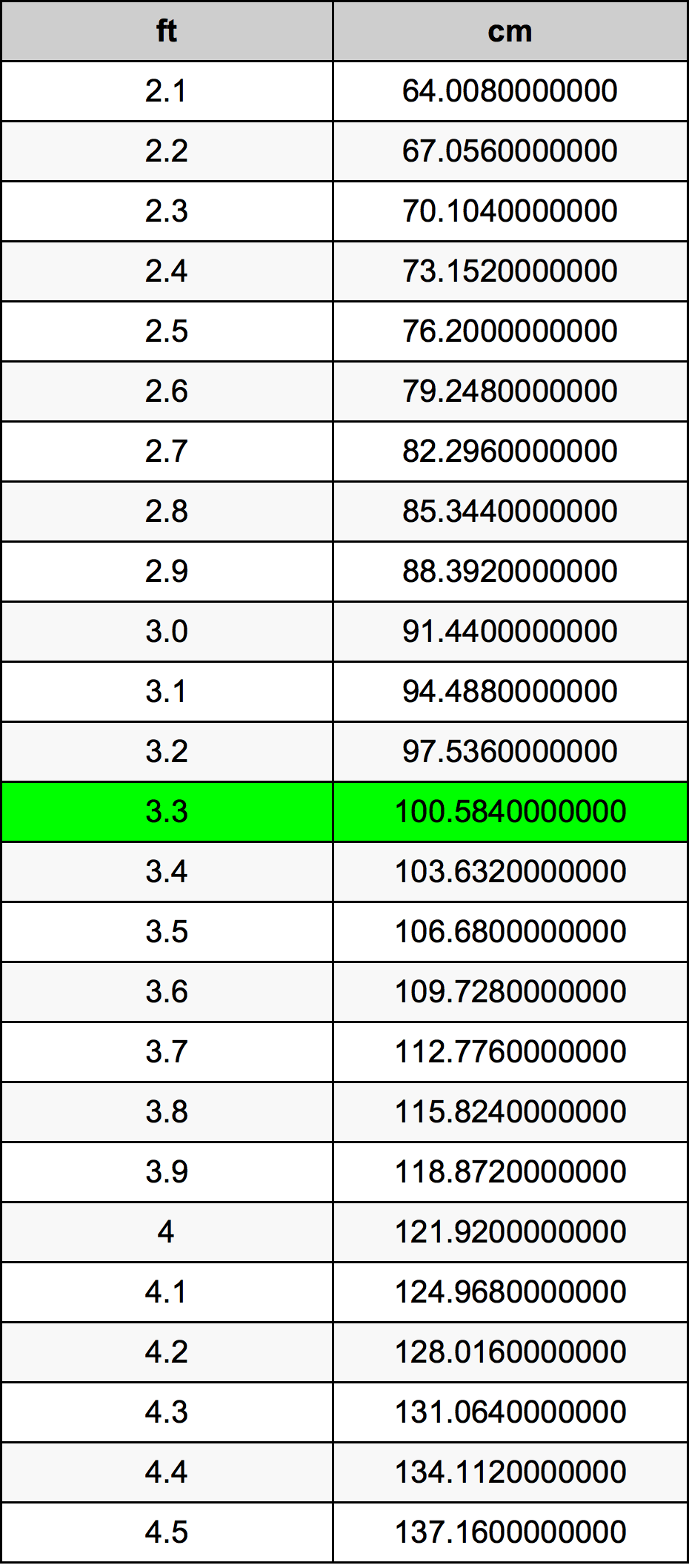Feet To Cm

# 3.3 ft to cm3.3 Feet to Centimeters

ft
=
cm

## How to convert 3.3 feet to centimeters?

 3.3 ft * 30.48 cm = 100.584 cm 1 ft
A common question is How many foot in 3.3 centimeter? And the answer is 0.1082677165 ft in 3.3 cm. Likewise the question how many centimeter in 3.3 foot has the answer of 100.584 cm in 3.3 ft.

## How much are 3.3 feet in centimeters?

3.3 feet equal 100.584 centimeters (3.3ft = 100.584cm). Converting 3.3 ft to cm is easy. Simply use our calculator above, or apply the formula to change the length 3.3 ft to cm.

## Convert 3.3 ft to common lengths

UnitLengths
Nanometer1005840000.0 nm
Micrometer1005840.0 µm
Millimeter1005.84 mm
Centimeter100.584 cm
Inch39.6 in
Foot3.3 ft
Yard1.1 yd
Meter1.00584 m
Kilometer0.00100584 km
Mile0.000625 mi
Nautical mile0.0005431102 nmi

## What is 3.3 feet in cm?

To convert 3.3 ft to cm multiply the length in feet by 30.48. The 3.3 ft in cm formula is [cm] = 3.3 * 30.48. Thus, for 3.3 feet in centimeter we get 100.584 cm.

## 3.3 Foot Conversion Table## Alternative spelling

3.3 ft to cm, 3.3 ft in cm, 3.3 Feet to cm, 3.3 Feet in cm, 3.3 Feet to Centimeter, 3.3 Feet in Centimeter, 3.3 ft to Centimeters, 3.3 ft in Centimeters, 3.3 ft to Centimeter, 3.3 ft in Centimeter, 3.3 Feet to Centimeters, 3.3 Feet in Centimeters, 3.3 Foot to Centimeters, 3.3 Foot in Centimeters